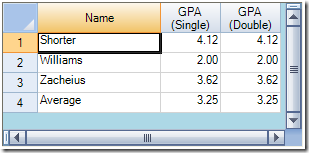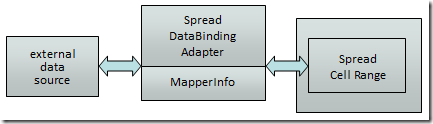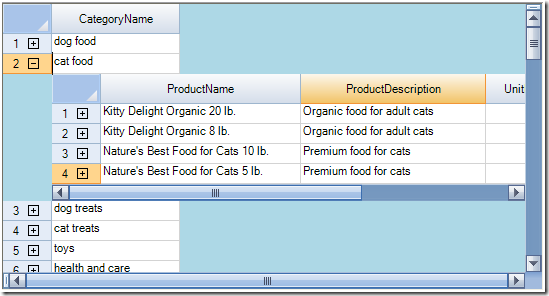// Turn off automatic column and field mapping.

// Bind the component to the data set.

// Set the fields for the columns.

fpSpread1.Sheets.Columns.DataField = "Price";

添加行或列到已绑定的表单// Bind the component to the data set.

fpSpread1.Sheets.AddUnboundRows(20, 1);// Bind the component to the data set.

fpSpread1.Sheets.AddColumns(20, 1);数据类型 单元格类型 布尔值 复选单元格 日期时间 日期时间单元格 双精度浮点值，单精度浮点值，十进制值 数值单元格 16位整型，32位整型，等等。 数据单元格 字符串 文本单元格 其它 通用单元格

FarPoint.Win.Spread.Data.SpreadDataBindingAdapter data = new FarPoint.Win.Spread.Data.SpreadDataBindingAdapter();

data.DataSource = dt;

data.SheetName = "Sheet1";

data.MapperInfo = new FarPoint.Win.Spread.Data.MapperInfo(3, 2, 1, 1);

data.FillSpreadDataByDataSource();数据类型 单元格类型 布尔值 复选单元格 日期时间 日期时间单元格 双精度浮点值，单精度浮点值，十进制值 数值单元格 16位整型，32位整型，等等。 数据单元格 字符串 文本单元格 其它 通用单元格

FarPoint.Win.Spread.Data.SpreadDataBindingAdapter data = new FarPoint.Win.Spread.Data.SpreadDataBindingAdapter();

data.DataSource = dt;

data.SheetName = "Sheet1";

data.MapperInfo = new FarPoint.Win.Spread.Data.MapperInfo(3, 2, 1, 1);

data.FillSpreadDataByDataSource();

使用层次化的数据显示• ChildRelationCount
• GetChildDataModel
• GetChildRelation
• GetChildSheets
• GetChildView
• GetChildVisible
• ParentRelationName

public class Score

{

private string classname;

public string ClassName

{

get { return classname; }

set { classname = value; }

}

{

set { grade = value; }

}

}

public class Student

{

private string name;

private string id;

private ArrayList score = new ArrayList();

public string Name

{

get { return name; }

set { name = value; }

}

public string Id

{

get { return id; }

set { id = value; }

}

public ArrayList Score

{

get { return score; }

}

}

private void Form1_Load(object sender, System.EventArgs e)

{

ArrayList list = new ArrayList();

Student s = new Student();

s.Name = "John Smith";

s.Id = "100001";

Score sc = new Score();

sc.ClassName = "math";

sc = new Score();

sc.ClassName = "English";

s = new Student();

s.Name = "David Black";

s.Id = "100002";

sc = new Score();

sc.ClassName = "math";

sc = new Score();

sc.ClassName = "English";

}

Spread for Windows Forms 5.0 中文版下载地址